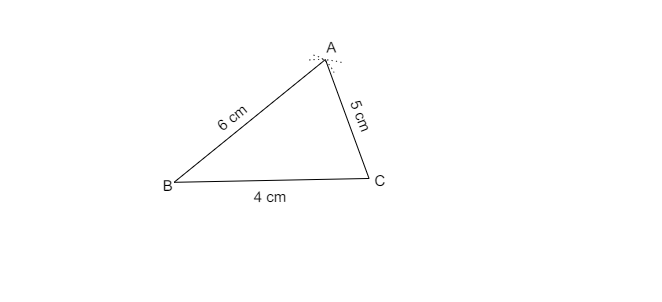# Construct a $\vartriangle ABC$ such that $AB=6$, $AC=5\ cm$ and the base $B C$ is $4\ cm$.

Given: $\vartriangle ABC$ such that $AB=6$, $AC=5\ cm$ and the base $BC$ is $4\ cm$.

To do: To construct $\vartriangle ABC$.

Solution:

1. Draw $BC=4\ cm$.

2. Take point $B$ as centre and radius$( 6\ cm)$, draw an arc.

3. Take point $C$ as centre and radius$( 5\ cm)$, draw an arc.

4. Both the arcs intersects at point $A$.$\vartriangle ABC$ is the required triangle.

Updated on: 10-Oct-2022

29 Views